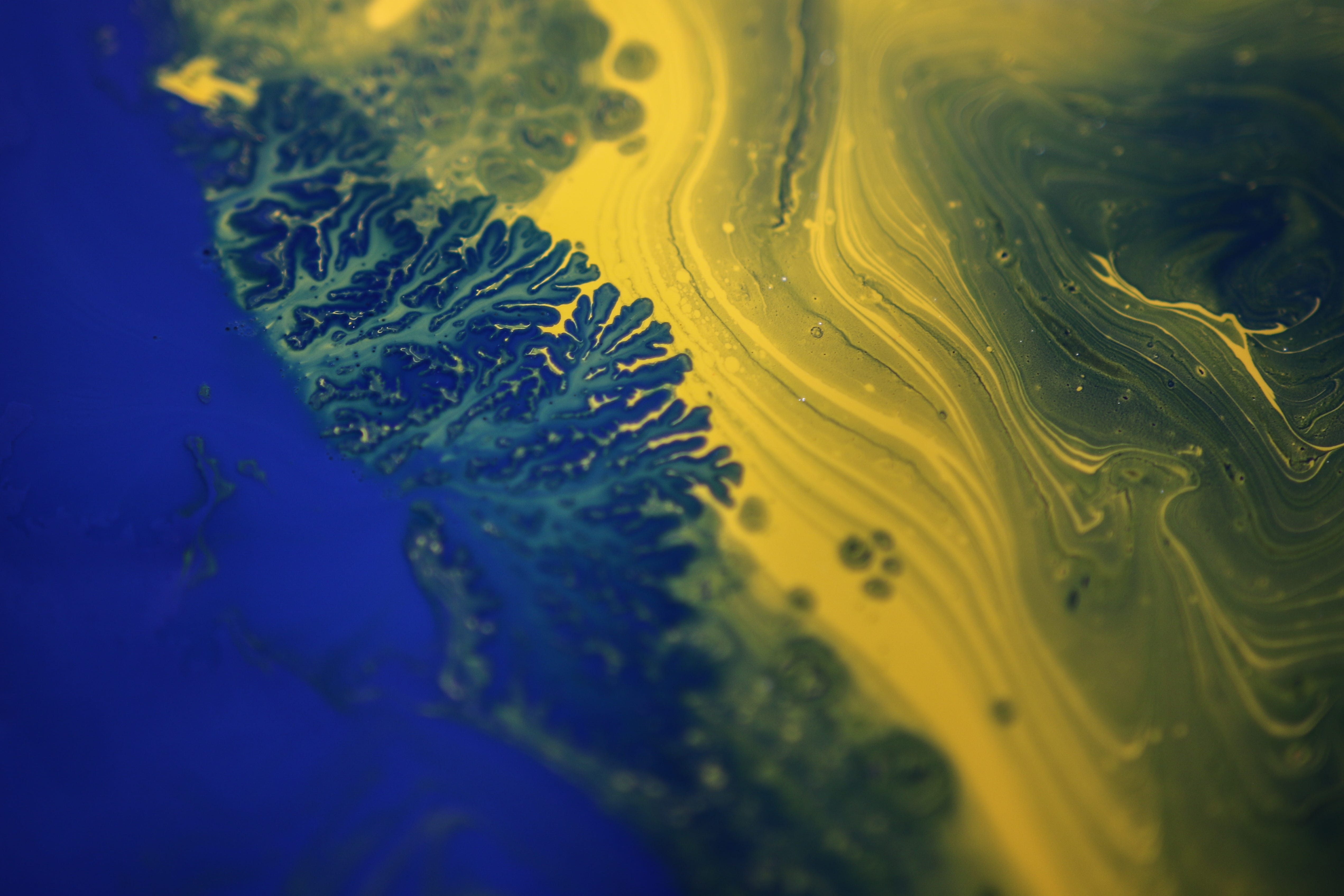# Programming Fractals in Python

## Learn how to create beautiful fractals through Python.Photo by Adrien Converse on Unsplash

# The Koch Snowflake

`import turtle`
`def koch_fract(turtle, divis, size):   if(divis == 0):      turtle.forward(size)   else:      for angle in [60, -120, 60, 0]:         koch_fract(turtle, divis - 1, size / 3)                        turtle.left(angle)`
`divis = 10 # any number of divisions, the larger the number, the more detailed the diagramsize = 2000 # any length`
`wn = turtle.Screen()wn.setup(width=1000, height=500)turtle.speed(100)turtle.pendown()`
`for i in range(0, 3):   koch_fract(turtle, divis, size)   turtle.left(-120)`

# The Barnsley Fern

`import matplotlib.pyplot as plt from random import randint`
`x = y = `
`for i in range(0, 50000):       p = randint(1, 100)           if p == 1:         x.append(0)         y.append(0.16*(y[i]))              if p >= 2 and p <= 86:         x.append(0.85*(x[i]) + 0.04*(y[i]))         y.append(-0.04*(x[i]) + 0.85*(y[i])+1.6)           if p >= 87 and p <= 93:         x.append(0.2*(x[i]) - 0.26*(y[i]))         y.append(0.23*(x[i]) + 0.22*(y[i])+1.6)               if p >= 94 and p <= 100:         x.append(-0.15*(x[i]) + 0.28*(y[i]))         y.append(0.26*(x[i]) + 0.24*(y[i])+0.44)`
`plt.scatter(x, y, s = 0.2, c ='#5dbb63') plt.axis("off")plt.show()`

# The Mandelbrot Set

`import numpy as npimport matplotlib.pyplot as plt`
`def mandelbrot(c, z):   global iterations   count = 0   for a in range(iterations):      z = z**2 + c      count += 1              if(abs(z) > 4):         break   return count`
`def mandelbrot_set(x, y):   m = np.zeros((len(x), len(y)))   for i in range(len(x)):      for j in range(len(y)):         c = complex(x[i], y[j])         z = complex(0, 0)         count = mandelbrot(c, z)         m[i, j] = count       return m`
`# creating our x and y arraysx = np.linspace(-2, 1, rows)y = np.linspace(-1, 1, cols)# create our mandelbrot setm = mandelbrot_set(x, y) # plot the set (best colors: binary, hot, bone, magma)plt.imshow(m.T, cmap = "magma")plt.axis("off")plt.show()`

## Nerd For Tech

From Confusion to Clarification

Written by

## Monique Cheng

A Filipino high-schooler studing in Singapore who likes to code. I literally just code whatever I want. Sometimes I also post random opinions :)

## More From Medium

Medium is an open platform where 170 million readers come to find insightful and dynamic thinking. Here, expert and undiscovered voices alike dive into the heart of any topic and bring new ideas to the surface. Learn more

Follow the writers, publications, and topics that matter to you, and you’ll see them on your homepage and in your inbox. Explore

If you have a story to tell, knowledge to share, or a perspective to offer — welcome home. It’s easy and free to post your thinking on any topic. Write on Medium